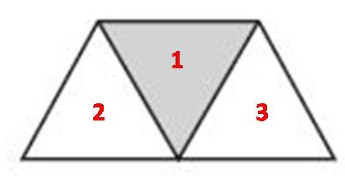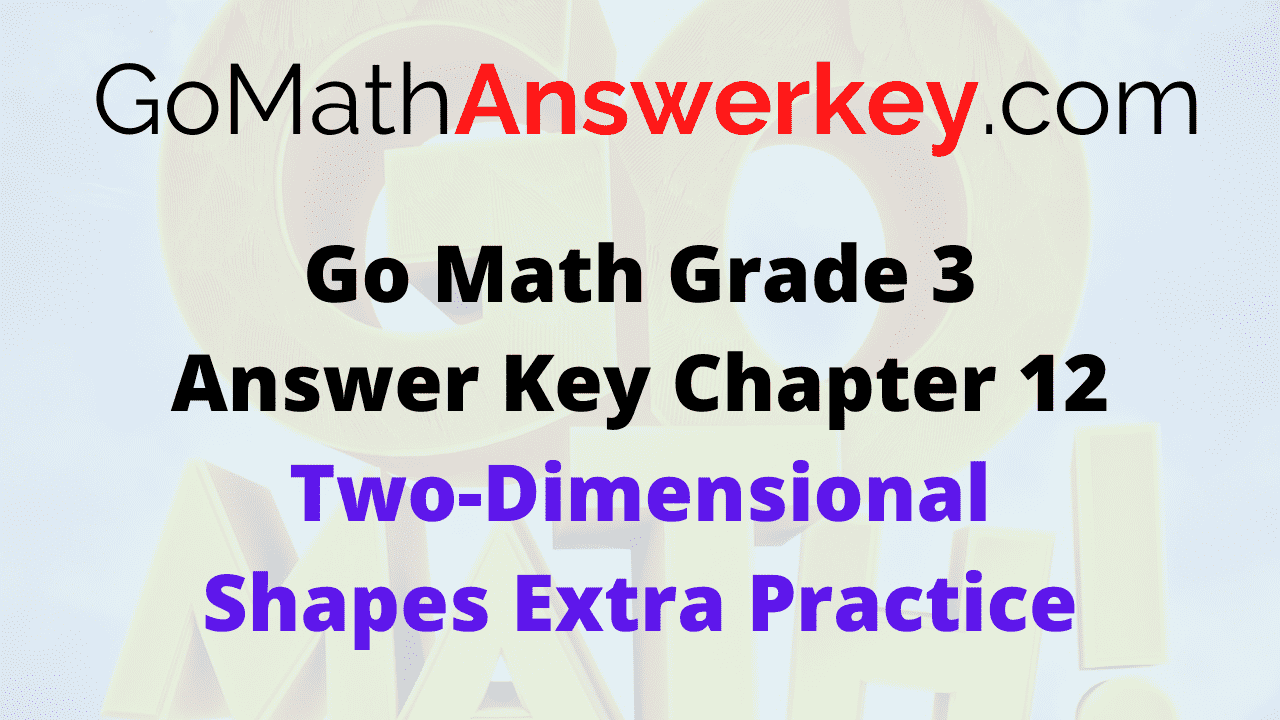# Go Math Grade 3 Answer Key Chapter 12 Two-Dimensional Shapes Extra Practice

Add extra skills for your students with Go Math Grade 3 Answer Key Chapter 12 Two-Dimensional Shapes Extra Practice. Elaborate your children thinking by solving every practice question on Go Math Grade 3 Chapter 12 Two-Dimensional Shapes Extra Practice. The Go Math Grade 3 Chapter 12 Two-Dimensional Shapes Extra Practice Answer Key is given for the guidance. See the creative approach to solve the math problems. HMH Go Math Grade 3 introducing a new way of problem-solving and providing the new path for the students to solve problems.

## Go Math Grade 3 Chapter 12 Two-Dimensional Shapes Extra Practice Answer Key

Download Go Math Grade 3 Answer Key Chapter 12 Two-Dimensional Shapes Extra Practice PDF and use it whenever you want. Solve all the problems with easy techniques and grab knowledge. Expert opinion is included to solve the problems of Grade 3 Chapter 12 Two-Dimensional Shapes Extra Practice. Follow the explanation and Go Math Grade 3 Chapter 12 Two-Dimensional Shapes Extra Practice Answer Key. The methodology implemented to solve problems is clear and easy.

### Common Core – Page No. 257000

Chapter 12 Extra Practice

Lessons 12.1–12.3

Name the polygon.

Question 1.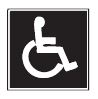_________

Explanation:Question 2._________

decagon

Explanation:10 sides; 10 angles; decagon

Question 3.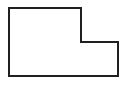_________

hexagon

Explanation: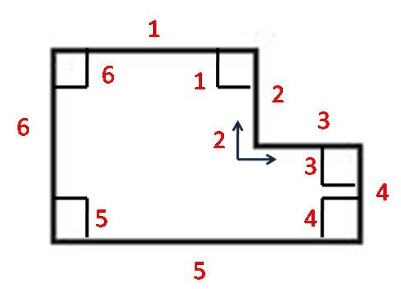6 sides; 6 angles; hexagon

Question 4._________

triangle

Explanation: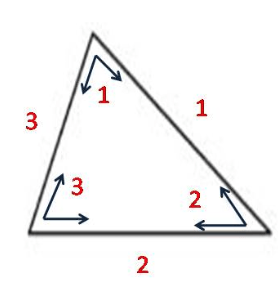3 sides; 3 angles; triangle

Question 5.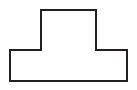_________

octagon

Explanation:8 sides; 8 angles; octagon

Question 6._________

pentagon

Explanation: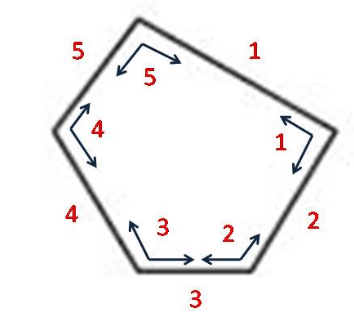5 sides; 5 angles; pentagon

Lesson 12.4

Look at the dashed sides of the polygon. Tell if they appear to be intersecting, perpendicular, or parallel. Write all the words that describe the sides.

Question 7._________
_________

perpendicular lines

Explanation:
The dashed sides are meeting to form a right angle. So, they are perpendicular lines.

Question 8.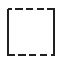_________

parallel lines

Explanation:
The dashed sides are not intersecting with each other. So, the given lines are parallel lines.

Question 9._________

intersecting lines

Explanation:
The dashed line segments meet and form an angle. So, they are intersecting lines.

Lesson 12.5

Circle all the words that describe the quadrilateral.

Question 10.Options:
a. rhombus
b. trapezoid
c. rectangle

c. rectangle

Explanation:
The given shape has two pairs opposite with the same length. Also, all the angles are right angles. The given shape is a rectangle.

Question 11.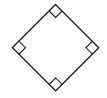Options:
a. square
b. rhombus
c. trapezoid

a. square
b. rhombus

Explanation:
The given shape has 4 sides with equal lengths. Also, all the angles are right angles. So, a possible answer is a square and rhombus.

Question 12.Options:
a. trapezoid
b. rectangle
c. rhombus

a. trapezoid

Explanation:
Even though the given shape has four sides, they are not equal. Also, it has only two right angles. The given shape is a trapezoid.

### Common Core – Page No. 258000

Lesson 12.6

Draw a quadrilateral that does not belong. Then explain why.

Question 1.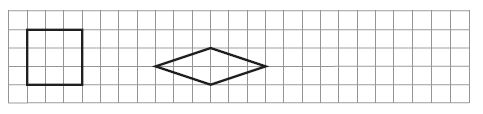Type below:
_________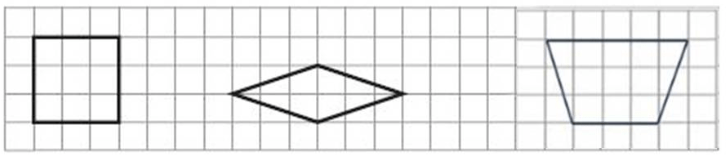Explanation:
The shape is a trapezoid. Even though the given shape has four sides, they are not equal. Also, the angles are not right angles.

Lesson 12.7

Use the triangles for 1–2. Write A, B, or C.
Then complete the sentences.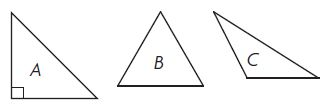Question 2.
Triangle ____ has 1 angle greater than a right angle and appears to have ____ sides of equal length.

Triangle C has 1 angle greater than a right angle and appears to have 0 sides of equal length.

Question 3.
Triangle____ has 1 right angle and appears to have ____ sides of equal length.

Triangle A has 1 right angle and appears to have 2 sides of equal length.

Lesson 12.8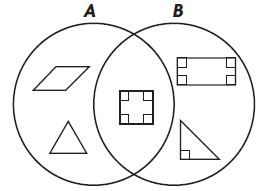Question 4.
What label could you use to describe Circle A?
Type below:
_________

All sides of Equal Lengths

Question 5.
What label could you use to describe Circle B?
Type below:
_________

Right Angle

Lesson 12.9

Draw lines to divide the shape into equal parts that show the fraction given.

Question 6.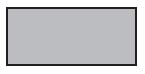$$\frac{1}{4}$$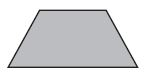$$\frac{1}{3}$$